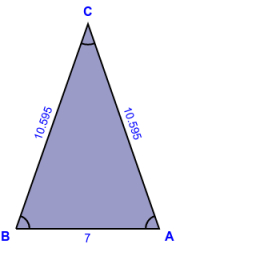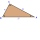# Area of a triangle

Find the area of a triangle with a base of 7 mm and a height of 10 mm?

A =  35 mm2

### Step-by-step explanation:

Try calculation via our triangle calculator.Did you find an error or inaccuracy? Feel free to write us. Thank you!Tips to related online calculators

#### You need to know the following knowledge to solve this word math problem:

We encourage you to watch this tutorial video on this math problem:

## Related math problems and questions:

• Height to the baseThe triangle area is 35 cm2. The size of the base is 10 cm. Find the length of height to the base.
• HeightThe content of the triangle is 35 cm2. The length of the base is 10 cm. Determine the length of the height on the base.
• Area of a triangleWhat is the area of a triangle that has the base 4 1/4 and the height of 3 3/3?
• Isosceles trapezoidFind the area of an isosceles trapezoid with bases of 8cm and 72mm. The height of the trapezoid is equal to three-quarters of the longer base.
• Triangular prismCalculate a triangular prism if it has a rectangular triangle base with a = 4cm and hypotenuse c = 50mm and height of the prism is 0.12 dm.
• CalculateCalculate the area of the ABE triangle AB = 38mm and height E = 42mm ps: please try a quick calculation
• Right triangleRight triangle legs has lengths 630 mm and 411 dm. Calculate the area of this triangle.
• Find areaFind area of a rectangle with a length of 58 foot and a width of 49 foot?
• Hexagonal pyramidFind the area of a shell of the regular hexagonal pyramid, if you know that its base edge is 5 cm long and the height of this pyramid is 10 cm.
• Triangular prismCalculate the volume and surface of the triangular prism ABCDEF with base of a isosceles triangle. Base's height is 16 cm, leg 10 cm, base height vc = 6 cm. The prism height is 9 cm.
• GlassHow many glass are needed to produce glass with base regular 5-gon if one base triangle in the base is 4.2 square cm and the height is 10 cm?
• A triangle 2A triangle has a base of 2 inches and a height of 2 3/4 inches. What is the area of the triangle?Find the height and surface of a regular quadrilateral pyramid with a base edge a = 8cm and a wall height w = 10cm. Sketch a picture.The base of vertical prism is an isosceles triangle whose base is 10 cm and the arm is 13 cm long. Prism height is three times the height of base triangle. Calculate the surface area of the prism.The regular triangular prism has a base in the shape of an isosceles triangle with a base of 86 mm and 6.4 cm arms, the height of the prism is 24 cm. Calculate its volume.The trapezium is formed by cutting the top of the right-angled isosceles triangle. The base of the trapezium is 10 cm and the top is 5 cm. Find the area of trapezium.The juice box has a volume of 200ml with its base is an isosceles triangle with sides a = 4,5cm and a height of 3,4cm. How tall is the box?